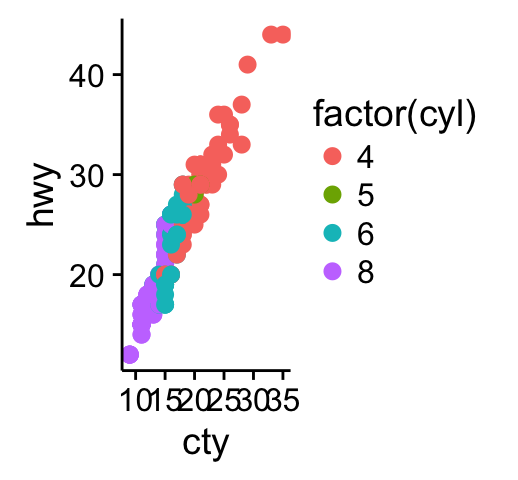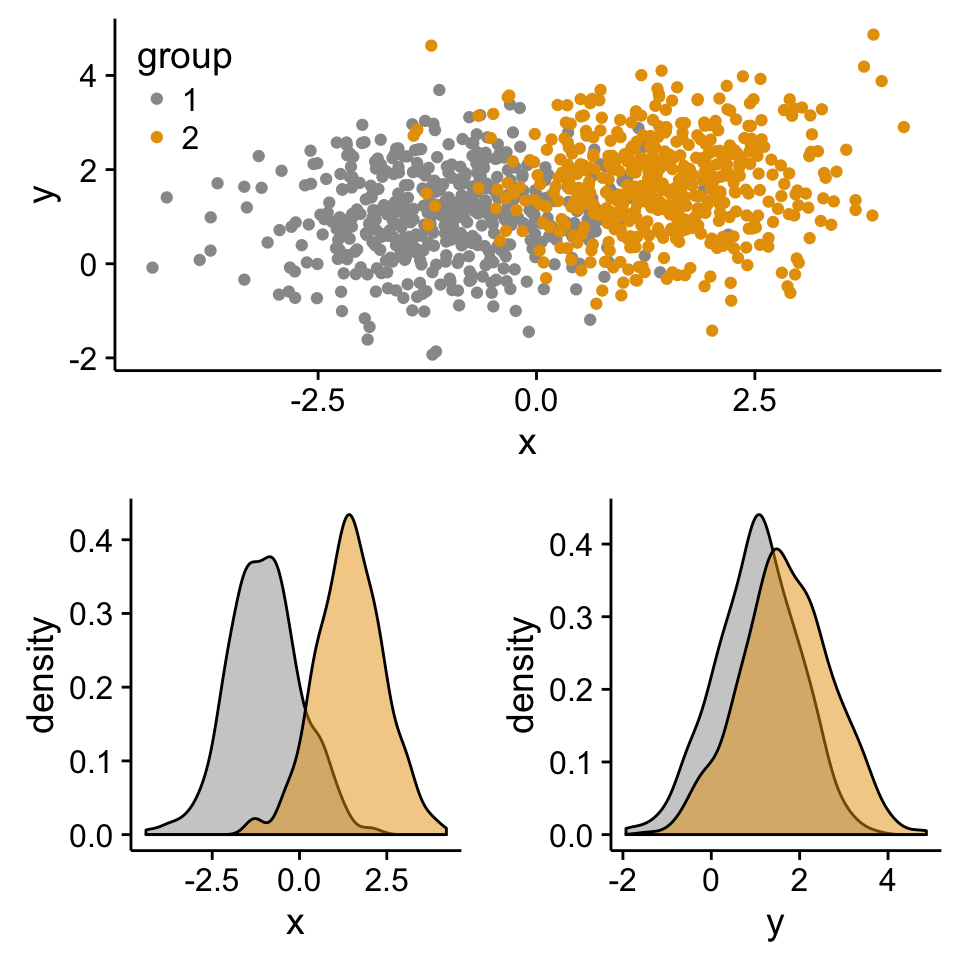Easy way to mix multiple graphs on the same page - R software and data visualization

## Prepare some data

The cowplot package is an extension to ggplot2 and it can be used to provide a publication-ready plots.

## Basic plotsRecall that, the function ggsave()[in ggplot2 package] can be used to save ggplots. However, when working with cowplot, the function save_plot() [in cowplot package] is preferred. It’s an alternative to ggsave with a better support for multi-figur plots.

## Arranging multiple graphs using cowplotCombine the two plots (the scatter plot and the bar plot):The function draw_plot() can be used to place graphs at particular locations with a particular sizes. The format of the function is:

• plot: the plot to place (ggplot2 or a gtable)
• x: The x location of the lower left corner of the plot.
• y: The y location of the lower left corner of the plot.
• width, height: the width and the height of the plot

• The function ggdraw() is used to initialize an empty drawing canvas.## grid.arrange: Create and arrange multiple plots

The R code below creates a box plot, a dot plot, a violin plot and a stripchart (jitter plot) :

Combine the plots using the function grid.arrange() [in gridExtra] :## Add a common legend for multiple ggplot2 graphs

This can be done in four simple steps :

1. Create the plots : p1, p2, ….
2. Save the legend of the plot p1 as an external graphical element (called a “grob” in Grid terminology)
3. Remove the legends from all plots
4. Draw all the plots with only one legend in the right panel

5. To save the legend of a ggplot, the helper function below can be used :

(The function above is derived from this forum. )## Scatter plot with marginal density plots

Step 1/3. Create some data :

Step 2/3. Create the plots :

Create a blank placeholder plot :

Step 3/3. Put the plots together:
Arrange ggplot2 with adapted height and width for each row and column :## Create a complex layout using the function viewport()

The different steps are :

1. Create plots : p1, p2, p3, ….
2. Move to a new page on a grid device using the function grid.newpage()
3. Create a layout 2X2 - number of columns = 2; number of rows = 2
4. Define a grid viewport : a rectangular region on a graphics device
5. Print a plot into the viewport

6.## Insert an external graphical element inside a ggplot

The function annotation_custom() [in ggplot2] can be used for adding tables, plots or other grid-based elements. The simplified format is :

• grob: the external graphical element to display
• xmin, xmax : x location in data coordinates (horizontal location)
• ymin, ymax : y location in data coordinates (vertical location)

• The different steps are :

1. Create a scatter plot of y = f(x)
2. Add, for example, the box plot of the variables x and y inside the scatter plot using the function annotation_custom()

3. As the inset box plot overlaps with some points, a transparent background is used for the box plots.

Create the graphs :If you have a solution to insert, at the same time, both p2_grob and p3_grob inside the scatter plot, please let me a comment. I got some errors trying to do this…

## Mix table, text and ggplot2 graphs

The functions below are required :

• tableGrob() [in the package gridExtra] : for adding a data table to a graphic device
• splitTextGrob() [in the package RGraphics] : for adding a text to a graph

• Make sure that the package RGraphics is installed.## Infos

This analysis has been performed using R software (ver. 3.1.2) and ggplot2 (ver. 1.0.0)
Contribution from ：http://www.sthda.com/english/wiki/ggplot2-easy-way-to-mix-multiple-graphs-on-the-same-page-r-software-and-data-visualization欢迎关注
0%